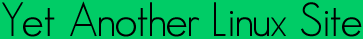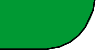Quick ?s
Cheat Sheets
Man Pages
The Lynx
Software
 ```TGAMMA(3) Linux Programmers Manual TGAMMA(3) NAME tgamma, tgammaf, tgammal - true gamma function SYNOPSIS #include double tgamma(double x); float tgammaf(float x); long double tgammal(long double x); Link with -lm. Feature Test Macro Requirements for glibc (see feature_test_macros(7)): tgamma(), tgammaf(), tgammal(): _XOPEN_SOURCE >= 600 || _ISOC99_SOURCE; or cc -std=c99 DESCRIPTION The Gamma function is defined by Gamma(x) = integral from 0 to infinity of t^(x-1) e^-t dt It is defined for every real number except for non-positive integers. For non-negative integral m one has Gamma(m+1) = m! and, more generally, for all x: Gamma(x+1) = x * Gamma(x) Furthermore, the following is valid for all values of x outside the poles: Gamma(x) * Gamma(1 - x) = PI / sin(PI * x) This function returns the value of the Gamma function for the argument x. It had to be called "true gamma function" since there is already a function gamma(3) that returns something else. ERRORS In order to check for errors, set errno to zero and call feclearex cept(FE_ALL_EXCEPT) before calling these functions. On return, if errno is non-zero or fetestexcept(FE_INVALID | FE_DIVBYZERO | FE_OVER FLOW | FE_UNDERFLOW) is non-zero, an error has occurred. A range error occurs if x is too large. A pole error occurs if x is zero. A domain error (or a pole error) occurs if x is a negative inte ger. CONFORMING TO C99. SEE ALSO gamma(3), lgamma(3) COLOPHON This page is part of release 3.05 of the Linux man-pages project. A description of the project, and information about reporting bugs, can be found at http://www.kernel.org/doc/man-pages/. GNU 2007-07-26 TGAMMA(3) ```Yals.net is © 1999-2009 Crescendo Communications Sharing tech info on the web for more than a decade! This page was generated Thu Apr 30 17:05:29 2009